## Chapter Outline:¶

1. Recap
2. Chapter Goals
3. Expectation-Maximization walkthrough
4. GMM application
5. Choosing Number of Components
6. GMM exploration
7. Conclusions
In :
%load_ext watermark
%watermark

from IPython.display import display
from IPython.core.debugger import set_trace as bp
# import standard libs
from pathlib import PurePath, Path
import sys
import time
import os
import pickle
os.environ['THEANO_FLAGS'] = 'device=cpu,floatX=float32'

# get project dir
pp = PurePath(Path.cwd()).parts[:-1]
pdir = PurePath(*pp)
data_dir = pdir/'data'
script_dir = pdir / 'scripts'
sys.path.append(script_dir.as_posix())

# import python scientific stack
import pandas as pd
pd.options.display.float_format = '{:,.4f}'.format
import numpy as np
import sklearn.mixture as mix
from sklearn.model_selection import TimeSeriesSplit
import statsmodels.tsa.api as smt
import statsmodels.api as sm
import scipy.stats as stats
from numba import jit
import math
import pymc3 as pm
from theano import shared, theano as tt
from multiprocessing import cpu_count

# import visual tools
from mpl_toolkits import mplot3d
import matplotlib as mpl
import matplotlib.pyplot as plt
import matplotlib.gridspec as gridspec
%matplotlib inline
import plotnine as pn
import mizani.breaks as mzb
import mizani.formatters as mzf
import seaborn as sns
savefig_kwds=dict(dpi=300, bbox_inches='tight')
# import util libs
from tqdm import tqdm
import warnings
warnings.filterwarnings("ignore")
from utils import cprint

# set globals
plt.style.use('seaborn-talk')
plt.style.use('bmh')
plt.rcParams['font.family'] = 'Bitstream Vera Sans'#'DejaVu Sans Mono'
#plt.rcParams['font.size'] = 9.5
plt.rcParams['font.weight'] = 'medium'
plt.rcParams['figure.figsize'] = 10,7

blue, green, red, purple, gold, teal = sns.color_palette('colorblind', 6)
RANDOM_STATE = 777

print()

2018-09-07T16:58:35-06:00

CPython 3.6.3
IPython 6.2.1

compiler   : GCC 7.2.0
system     : Linux
release    : 4.15.0-33-generic
machine    : x86_64
processor  : x86_64
CPU cores  : 12
interpreter: 64bit

/media/bcr/HDD/anaconda3/envs/bayes_dash/lib/python3.6/site-packages/h5py/__init__.py:36: FutureWarning: Conversion of the second argument of issubdtype from float to np.floating is deprecated. In future, it will be treated as np.float64 == np.dtype(float).type.
from ._conv import register_converters as _register_converters

pandas 0.23.3
numpy 1.14.5
sklearn 0.19.1
statsmodels 0.9.0
scipy 1.1.0
pymc3 3.5
matplotlib 2.2.2
seaborn 0.9.0
plotnine 0.3.0


## Recap¶

In the last chapter we went through some foundational concepts.

We discussed stationarity, why it is important, and its rules. We were able to understand the concept by thoroughly examining the most common ways that financial time series violate the requirements.

We illustrated how financial time series have trends (means vary with time), changing volatility (variance changes with time), clustering volatility (autocorrelation between t and t+i).

Using statistical techniques we demonstrated that asset returns from different time periods and scales appear to come from different distributions.

We concluded that for our purposes of predicting future return distributions, techniques that cannot accomodate the time varying properties of financial asset returns are a danger to our trading acocunts.

## Chapter Goals¶

In this chapter we will see how Gaussian Mixture Models (gmm) can help overcome some of the time series prediction issues we identified in chapter 1.

1. Understand the intuition behind how GMM's can approximate nonstationary distributions
2. Understanding the underlying Expectation-Maximization algorithm
3. Use sklearn to choose the optimal number of components
4. Use seaborn, plotnine, and matplotlib for visual analysis

## Gaussian Mixture Models (GMM) Intuition¶

Our biggest pain point results from the idea that asset returns are comprised of multiple distributions. Time varying means, and volatilities can be considered as coming from different distributions, regimes, or states (moving forward I will use those terms interchangeably). Each regime has its own parameters.

For example consider two regimes with the following characteristics.

1. Stable, low volatility
2. Risky, high(er) volatility



We can make an assumption that every data point from our return series has come from either the stable or risky regime. If we can classify each data point correctly then we can use the current regime's parameters as the input to our prediction for the next period. As we know, the best estimate for an unpredictable future state is the current state.

That sounds good however we still have some challenges.

1. We do not know the parameters, ($\mu, \sigma$), for the two regimes.
2. We do not know which datapoint came from which regime.

On first pass this problem seems intractable.

Fortunately for us, some smart people devised a solution.

Expectation-maximization algorithm (EM, E-M, em, etc.)

Most introductory texts on this subject explain the topic using heavy maths first, somewhat obscuring the concept and leaving one feeling like its magic and moving on. I hope to avoid that outcome in this brief intro and make it more intuitive.

### Why do we need Expectation-Maximization, why bother to understand it?¶

The EM algorithm and derivations thereof underpin many unsupervised learning methods including mixture modeling. It is useful in many real world applications where:

- Data is corrupted.
- Data is missing.
- We do not know the parameters of data generating process (aka model, distribution).
- We do not know which data generating process generated which data point.

### Expectation-Maximization Walkthrough Example¶

Let's continue with our example of an asset return series being generated by a combination of 2 Gaussian distributions. To start we let's say we have 3 trading years (252*3) worth of return data.

To start the algorithm all we have to do is guess at the parameters even though we know those guesses are likely incorrect.

For example we can assume the stable regime has returns with mean 2.5% and sigma 9%, while the risky regime has -1.0% returns and 25% volatility. Furthermore we will assume that each regime occurs with equal probability.

The next step is to assume those incorrect guesses are correct and proceed to assign responsiblities (aka probabilities or weights) to each data point. So for example assume the first data point we have is a return of 1.3%. We must compute the probability that the stable regime, a Gaussian distribution with mean 2.5% and std of 9% generated that return. We do that for all the returns on the first pass, again reusing those initial incorrect guesses about the means and volatilties. We then compute the probability that the risky regime generated those data points.

Next we sum those probabilities, normalize them, and use those assignments to reestimate the means and volatilities of the regimes. Rinse and repeat.

What's remarkable about this iterative process is that we are guaranteed to improve our estimate at each iteration.

Note: this algorithm does not guarantee a global solution, but rather a local one. In practice the algorithm is started with multiple random parameter initializations in order to recover the best estimates of the true parameters.

For this example let's assume we have deduced the true means, sigmas, and prior probabilities for each of the distributions, and we want to test the ability of the EM algorithm to recover this information from noisy data.

To set up this brief demonstration below we define the number of samples n, the true means, and sigmas, as well as the true prior probability of each regime.

#### Create Synthetic Return Data¶

In :
# Let's create some example return data to walk through the process. We will create a synthetic return series composed of two gaussians with different parameters.
n = 252 * 7

true_stable_mu, true_stable_sigma = 0.15, 0.25
true_risky_mu, true_risky_sigma = -0.17, 0.45

true_prob_stable = 0.65
true_prob_risky = 1 - true_prob_stable

true_mus = np.array([true_stable_mu, true_risky_mu])
true_sigmas = np.array([true_stable_sigma, true_risky_sigma])
true_probs = np.array([true_prob_stable, true_prob_risky])


Then we create a little helper fun that takes our true parameters and creates a noisy, synthetic (fake) return series by mixing our true distributions.

In :
def mix_data(mus, sigmas, probs, n):

np.random.seed(0)
# randomly sample from binomial to select distr.
z = np.random.binomial(1, true_probs, n)
# sample from normal distr. and associated parameters according to z
X = np.random.normal(true_mus[z], true_sigmas[z])

# fake dates to make it look real
fake_dates = pd.date_range('2011', periods=n)
fake_returns = pd.Series(X, index=fake_dates)

return fake_returns

mixed = mix_data(true_mus, true_sigmas, true_probs, n=n)

fig, axs = plt.subplots(nrows=3, figsize=(10,7))#, sharex=True)

mixed.plot(ax=axs, label='fake returns')
mixed.cumsum().plot(ax=axs, label='fake cumulative returns')
sns.distplot(mixed, ax=axs, kde_kws=dict(cut=0), label='fake kde returns')
for ax in axs:
ax.legend(loc='upper left')
ax.tick_params('both', direction='inout', length=7, width=1, which='major')
plt.tight_layout()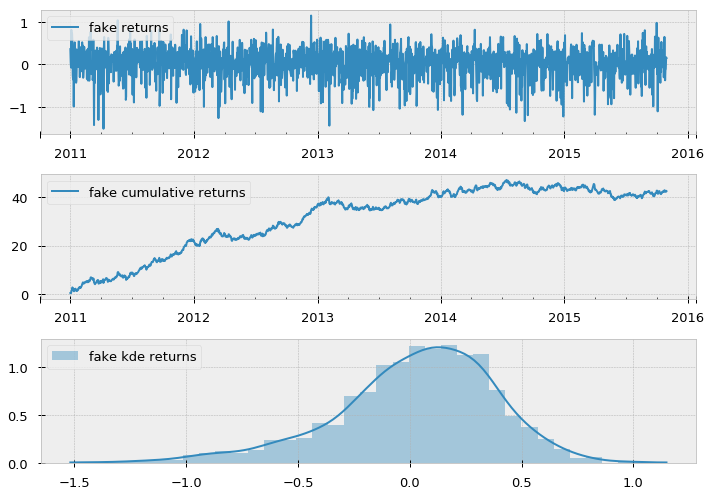#### Code Normal Distribution Class¶

After creating our somewhat realistic looking mixture we need to code our normal distribution class.

It needs to be able to take mu, sigma parameters and contain methods to compute the log probability density function (logpdf, or log_density) of the data given the parameters. It also needs to be able to estimate the parameters of the normal distribution given the data and weights.

In :
# code adapted from: https://github.com/sseemayer/mixem

class Normal:
"""Univariate normal distribution with parameters (mu, sigma)."""

def __init__(self, mu, sigma):
self.mu = mu
self.sigma = sigma

def log_density(self, data):
"""fn: compute log pdf of normal distr. given parameters and data"""

assert(len(data.shape) == 1), "Expect 1D data!"

# uncomment to confirm they produce same values
log_pdf = stats.norm.logpdf(data, loc=self.mu, scale=self.sigma)
#log_pdf = - (data - self.mu) ** 2 / (2 * self.sigma ** 2) - np.log(self.sigma) - 0.5 * np.log(2 * np.pi)
return log_pdf

def estimate_parameters(self, data, weights):
"""fn: estimate parameters of normal distr. given data and weights"""

assert(len(data.shape) == 1), "Expect 1D data!"

wsum = np.sum(weights)

self.mu = np.sum(weights * data) / wsum
self.sigma = np.sqrt(np.sum(weights * (data - self.mu) ** 2) / wsum)


#### Initialize Expectation-Maximization Algorithm¶

Now we can make incorrect guesses about the parameters of the two distributions as initial starting points to the EM algorithm.

In :
# terrible guesses at the true prior probability
init_stable_prob = 0.5
init_volatile_prob = 0.5

# guesses at starting mean
init_stable_mean = 0.10
init_volatile_mean = -0.1

# guesses at starting std
init_stable_std = 0.10
init_volatile_std = 0.3

init_probs = np.array([init_stable_prob, init_volatile_prob])
init_means = np.array([init_stable_mean, init_volatile_mean])
init_sigmas = np.array([init_stable_std, init_volatile_std])


Now we are ready to code the actual algorithm steps. Code is adapted from here

In :
# wrap our distributions in a list
distributions = [Normal(init_means, init_sigmas),
Normal(init_means, init_sigmas)]
# set data
data = mixed.copy()

# set algorithm parameters
max_iterations = tol_iters = 1000
tol=1e-5

# get key dim info
n_distr = true_mus.shape
n_data = data.shape

weight = np.array(init_probs) # init weight array
last_ll = np.full((tol_iters, ), np.nan) # init log-likelihood array
resp = np.empty((n_data, n_distr)) # init algo weights/resp array
log_density = np.empty((n_data, n_distr)) # init logpdf array

iteration = 0 # init counter


#### Run Expectation-Maximization Algorithm¶

In :
while True:
# ---------------------------------------------------------
# E-step
# ---------------------------------------------------------

# compute responsibilities aka weights
for d in range(n_distr):
log_density[:, d] = distributions[d].log_density(data)

# normalize responsibilities of distributions so they sum up to one for example
resp = weight[np.newaxis, :] * np.exp(log_density)
resp /= np.sum(resp, axis=1)[:, np.newaxis]

# compute log-likelihood
log_likelihood = np.sum(resp @ log_density.T) # matrix multiplication

# ---------------------------------------------------------
# M-step
# ---------------------------------------------------------

# now that we have the new weights we update the parameters
# of the distributions
for d in range(n_distr):
distributions[d].estimate_parameters(data, resp[:, d])

weight = np.mean(resp, axis=0)

# ---------------------------------------------------------
# check convergence
# ---------------------------------------------------------

if np.isnan(log_likelihood):
last_ll = log_likelihood
print('loglk is nan')
break

if  ((last_ll - log_likelihood) / last_ll) <= tol:
last_ll = log_likelihood
print('change in loglk less than tolerance')
break

if iteration >= max_iterations:
last_ll = log_likelihood
print('reached maximum iterations')
break

# ---------------------------------------------------------
# store value of current iteration in last_ll
#   and shift older values to the right
# ---------------------------------------------------------
last_ll[1:] = last_ll[:-1]
last_ll = log_likelihood

# ---------------------------------------------------------
# info display
# ---------------------------------------------------------
mus = np.array([distributions[i].mu for i in range(n_distr)])
sigs = np.array([distributions[i].sigma for i in range(n_distr)])

regime_map = {0:'stable', 1:'risky'}
iter_data = (pd.DataFrame(np.vstack([mus, sigs, weight,
true_mus, true_sigmas, true_probs]),
columns=[f'{regime_map[i]} regime' for i in range(n_distr)],
index=['means', 'sigmas', 'weights',
'true_means', 'true_sigmas', 'true_weights'])
.round(3))

if iteration % 50==0:
print()
print('-'*77)
print(f'iteration: {iteration}')
print(f"ll new: {last_ll.round(3)}")
display(iter_data.T)
iteration += 1

-----------------------------------------------------------------------------
iteration: 0
ll new: -7322372.071

means sigmas weights true_means true_sigmas true_weights
stable regime 0.1250 0.1280 0.3600 0.1500 0.2500 0.6500
risky regime -0.0330 0.4360 0.6400 -0.1700 0.4500 0.3500
-----------------------------------------------------------------------------
iteration: 50
ll new: -2192438.086

means sigmas weights true_means true_sigmas true_weights
stable regime 0.1490 0.2330 0.5770 0.1500 0.2500 0.6500
risky regime -0.1460 0.4380 0.4230 -0.1700 0.4500 0.3500
-----------------------------------------------------------------------------
iteration: 100
ll new: -2093505.191

means sigmas weights true_means true_sigmas true_weights
stable regime 0.1400 0.2460 0.6530 0.1500 0.2500 0.6500
risky regime -0.1930 0.4450 0.3470 -0.1700 0.4500 0.3500
-----------------------------------------------------------------------------
iteration: 150
ll new: -2070105.946

means sigmas weights true_means true_sigmas true_weights
stable regime 0.1380 0.2500 0.6730 0.1500 0.2500 0.6500
risky regime -0.2090 0.4460 0.3270 -0.1700 0.4500 0.3500
-----------------------------------------------------------------------------
iteration: 200
ll new: -2063535.044

means sigmas weights true_means true_sigmas true_weights
stable regime 0.1370 0.2510 0.6790 0.1500 0.2500 0.6500
risky regime -0.2140 0.4460 0.3210 -0.1700 0.4500 0.3500
-----------------------------------------------------------------------------
iteration: 250
ll new: -2061503.148

means sigmas weights true_means true_sigmas true_weights
stable regime 0.1370 0.2510 0.6810 0.1500 0.2500 0.6500
risky regime -0.2160 0.4460 0.3190 -0.1700 0.4500 0.3500
change in loglk less than tolerance

In :
_last_ll = last_ll[pd.notnull(last_ll)]
plt.plot(np.arange(0,len(_last_ll)), _last_ll[::-1])
plt.title('Maximizing Log Likelihood')

Out:
Text(0.5,1,'Maximizing Log Likelihood')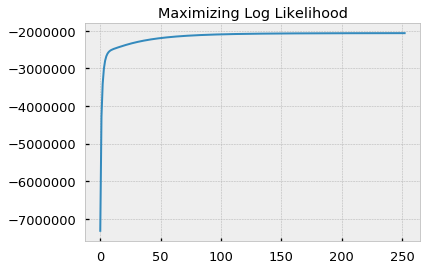Not bad right? This algorithm is pretty flexible although you can adjust the true parameters and/or the guesses and you will find some of the limitations. However how those are addressed are beyond the scope of this chapter.

My goal was to provide a simple working example of the algorithm, in order for us to gain a deeper understanding of what is happening inside the black box of the algorithm.

Now we can move forward.

## Fitting Mixture Models Using Real Data¶

In :
infp = PurePath(data_dir/'etf_returns_2004-11-19-2017-12-31.parq')
.assign(year=lambda df: df.index.year)) # add year column for later conv.
cprint(R)

-------------------------------------------------------------------------------
dataframe information
-------------------------------------------------------------------------------
SPY     QQQ     TLT    GLD     EFA     EEM  year
Date
2017-12-25  0.0000  0.0000  0.0000 0.0000  0.0000  0.0000  2017
2017-12-26 -0.0012 -0.0054  0.0030 0.0068 -0.0003 -0.0009  2017
2017-12-27  0.0005  0.0001  0.0129 0.0038  0.0019  0.0026  2017
2017-12-28  0.0021  0.0012 -0.0009 0.0051  0.0006  0.0068  2017
2017-12-29 -0.0038 -0.0062  0.0016 0.0065  0.0007  0.0047  2017
--------------------------------------------------
<class 'pandas.core.frame.DataFrame'>
DatetimeIndex: 3421 entries, 2004-11-19 to 2017-12-29
Data columns (total 7 columns):
SPY     3421 non-null float64
QQQ     3421 non-null float64
TLT     3421 non-null float64
GLD     3421 non-null float64
EFA     3421 non-null float64
EEM     3421 non-null float64
year    3421 non-null int64
dtypes: float64(6), int64(1)
memory usage: 213.8 KB
None
-------------------------------------------------------------------------------


In :
sym = 'SPY' # example symbol
df = R.loc['2005':].copy() # use 2005 cutoff b/c it's first full year of data
df.info()

<class 'pandas.core.frame.DataFrame'>
DatetimeIndex: 3390 entries, 2005-01-03 to 2017-12-29
Data columns (total 7 columns):
SPY     3390 non-null float64
QQQ     3390 non-null float64
TLT     3390 non-null float64
GLD     3390 non-null float64
EFA     3390 non-null float64
EEM     3390 non-null float64
year    3390 non-null int64
dtypes: float64(6), int64(1)
memory usage: 211.9 KB

In :
df2 = (df[[sym]]
.assign(normal=lambda df:
stats.norm.rvs(df[sym].mean(), df[sym].std(),
size=len(df[sym])))
.assign(laplace=lambda df:
stats.laplace.rvs(df[sym].mean(), df[sym].std(),
size=len(df[sym]))))


Out:
SPY normal laplace
Date
2005-01-03 -0.0047 0.0067 0.0329
2005-01-04 -0.0123 -0.0092 -0.0065
2005-01-05 -0.0069 0.0029 0.0051
2005-01-06 0.0051 -0.0036 0.0138
2005-01-07 -0.0014 -0.0125 0.0248
In :
p = (pn.ggplot(pd.melt(df2), pn.aes(x='value', color='variable'))
+pn.geom_density(pn.aes(fill='variable'), alpha=0.5))
p.draw();

p = (pn.ggplot(pd.melt(df2), pn.aes(x='value', color='variable'))
+pn.geom_histogram(pn.aes(y='..ndensity..', fill='variable'), alpha=0.5))
p.draw();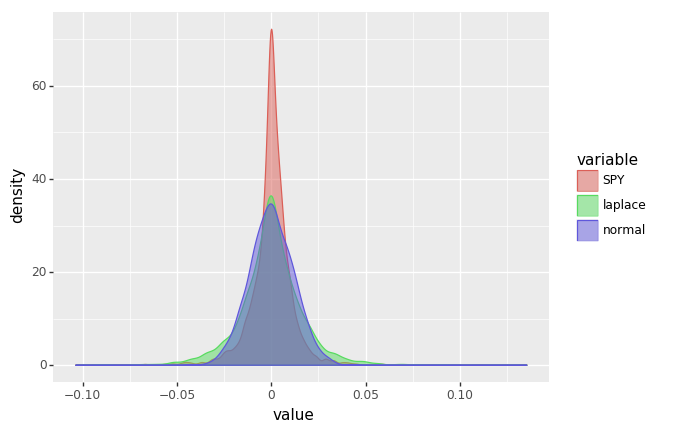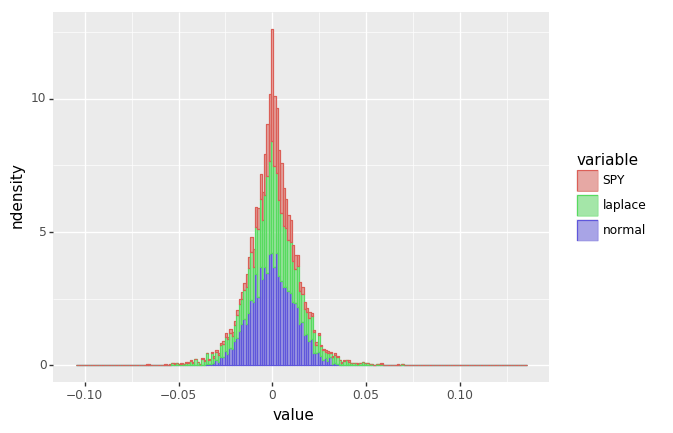Continuing with our example, using SPY returns, let's use sklearn to fit a GMM model. First we will fit one using a single component. This is equivalent to fitting a single normal distribution to the set of returns.

In :
def make_gmm(n_components, max_iter=150, random_state=RANDOM_STATE):
"""fn: create gmm object"""
model_kwds = dict(n_components=n_components,
max_iter=max_iter,
n_init=100,
random_state=RANDOM_STATE)

gmm = mix.GaussianMixture(**model_kwds)
return gmm

def print_gmm_results(gmm, X):
print('-'*25)
print(f'means: {gmm.means_.ravel()}')
print('-'*25)
print(f'covars: {gmm.covariances_.ravel()}')
print('-'*25)
print(f'sqrt covar: {np.sqrt(gmm.covariances_.ravel())}')
print('-'*25)
print(f'aic: {gmm.aic(X):.5f}')
print(f'bic: {gmm.bic(X):.5f}')
print('-'*25)

#######
s = df[sym]
max_iter = 150
_X = s.values.reshape(-1, 1)

gmm1 = make_gmm(1, max_iter)
gmm1.fit(_X)

preds = gmm1.predict(_X)

print_gmm_results(gmm1,_X)
print(f'data derived mean: {s.mean():.7f}')
print(f'data derived std: {s.std():.7f}')

-------------------------
means: [0.00023363]
-------------------------
covars: [0.00013651]
-------------------------
sqrt covar: [0.01168375]
-------------------------
aic: -20568.42188
bic: -20556.16471
-------------------------
data derived mean: 0.0002336
data derived std: 0.0116426


### Comparing AIC and BIC of Different numbers of Component Mixtures¶

Note that the mean and standard deviation is the same as computed in the previous plot. Also note the aic and bic metrics. These reference the Akaike Information Criterion and the Bayesian Information Criterion. Without delving too heavily into the theoretical pros and cons of each, we can just say they are both methods which allow us to compare the relative suitability of different models. Generally speaking, when choosing among a set of models we want to choose the aic or bic with the smallest information criterion value.

AIC rewards goodness of fit (as assessed by the likelihood function), but it also includes a penalty that is an increasing function of the number of estimated parameters. The penalty discourages overfitting, because increasing the number of parameters in the model almost always improves the goodness of the fit. - wikipedia

The importance of the penalty component in each of the ICs cannot be overstated. As always, in the limit, you could technically fit a distribution, or add a parameter for every datapoint, thereby grossly overfitting the model.

Both metrics implement a penalty, however the bic penalizes additional parameters more heavily than the aic and will always result in a selecting fewer parameters than the aic would. Let's walkthrough a quick demo of this.

In :
gmm2 = make_gmm(2, max_iter)
gmm2.fit(_X)

print_gmm_results(gmm2,_X)

-------------------------
means: [-0.00266647  0.00078149]
-------------------------
covars: [6.15239699e-04 4.41844483e-05]
-------------------------
sqrt covar: [0.02480403 0.00664714]
-------------------------
aic: -21866.06618
bic: -21835.42325
-------------------------


Above we fit a GMM model with 2 components. Notice that both the aic and bic are smaller than their single component counterparts. This implies that the model with 2 components is "better" than the one with a single component. What about more components? Let's try 15.

In :
gmm15 = make_gmm(15, max_iter)
gmm15.fit(_X)

print_gmm_results(gmm15,_X)

-------------------------
means: [ 0.01388283  0.00023159 -0.02183881  0.03311002 -0.00824004  0.00862988
-0.03084074  0.12304543 -0.07724461  0.00415294 -0.00324585 -0.01365624
0.02042235  0.060776   -0.04614129]
-------------------------
covars: [7.99686450e-06 4.39321022e-06 1.23421269e-05 3.91651572e-05
6.51551472e-06 6.34283435e-06 1.69453159e-05 1.58093460e-04
2.21893063e-04 5.60964597e-06 5.89996895e-06 7.85232708e-06
1.14285799e-05 3.71869515e-05 2.97916425e-05]
-------------------------
sqrt covar: [0.00282787 0.002096   0.00351314 0.00625821 0.00255255 0.0025185
0.00411647 0.01257352 0.01489608 0.00236847 0.00242899 0.0028022
0.00338062 0.00609811 0.00545817]
-------------------------
aic: -22051.87240
bic: -21782.21465
-------------------------


Now we see an example where the aic has improved by getting smaller but the bic is actually larger than the 2 component model. Here we see the result of the bic penalizing additional parameters more heavily.

### Choosing The Optimal Number of Components using AIC/BIC¶

Below we can see a comparison between the two metrics as we increase the number of components. We can also see that they choose different numbers of optimal components.

In :
def make_ic_series(list_of_tups, name=None):
"""fn: convert list of tuples for
information criterion (aic, bic) into series
# args
list_of_tups : list() of tuples()
tuple is n_component, tuple is IC
name : str(), name of IC

# returns
s : pd.Series()
index is n_components, values are IC's
"""
s = (pd.DataFrame(list_of_tups)
.rename(columns={0:'n_components', 1:name})
.set_index('n_components')
.squeeze())
return s

In :
n_components = np.arange(1,15)
aics = []
bics = []

for n in n_components:
tmp_gmm = make_gmm(n, max_iter).fit(_X)
aics.append((n, tmp_gmm.aic(_X)))
bics.append((n, tmp_gmm.bic(_X)))

bics = make_ic_series(bics, 'bic')
aics = make_ic_series(aics, 'aic')

plt.figure(figsize=(10,7))
plt.plot(n_components, bics.values, color=blue, label=f'BIC min = {np.argmin(bics)}')
plt.axvline(np.argmin(bics), color=blue)

plt.plot(n_components, aics.values, color=red, label=f'AIC min = {np.argmin(aics)}')
plt.axvline(np.argmin(aics), color=red)

plt.legend(loc='best')
plt.xlabel('n_components');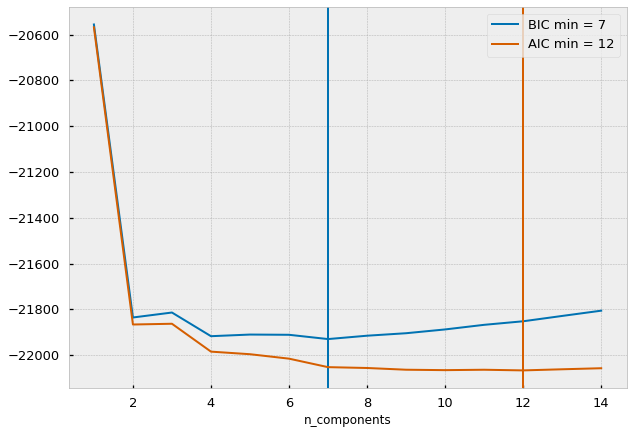As shown above the aic selects 12 components as being the best model whereas the bic selects only 7. For convenience we will use the bic recommendation for the remainder of the this notebook. One exercise I leave for the reader is to run the aic, bic, component analysis using different asset returns, and using different lookback periods.

## Visualizing Regimes¶

Below is a demonstration of the how varied the components are across multiple lookback periods. Note that we choose only 2 components even though the BIC informed us that 7 was the "optimal" number. This is done for interpretability purposes. In this example one regime will be considered stable and the other as risky. Due to the unsupervised nature of the algorithm we cannot know which regime is which apriori. So for the rest of the example the regimes will be named s1, s2 as in state 1, and state 2.

Note what we make use of the TimeSeriesSplit tool from sklearn to implement our walkforward testing.

In :
%%time
np.random.seed(0)

lookback = 252 #* 3 # 3 trading years
n_components = 2
max_iter = 1000
n_split = n_components * 4
grid_rows = math.ceil(n_split/2)

# stash data in lists
preds = []
pred_means = []
pred_covs = []

### begin plot code ###
fig = plt.figure(figsize=(15,20))
outer_grid = gridspec.GridSpec(grid_rows, 2)
colors = plt.cm.rainbow(np.linspace(0, 1, n_components))

tscv = TimeSeriesSplit(n_splits=n_split, max_train_size=lookback)

for i, (train, test) in enumerate(tscv.split(s)):
tmp_train = s.iloc[train] # temporary train data
tmp_test = s.iloc[test] # temporary test data

_X = tmp_train.values.reshape(-1,1) # format pd.Series for sklearn
gmm = make_gmm(n_components, max_iter) # make model
gmm.fit(_X) # fit model

# predict hidden states
hidden_states = gmm.predict(_X)

# store output in lists
preds.append(hidden_states)
pred_means.append(gmm.means_)
pred_covs.append(gmm.covariances_)

# make inner grid for subplots
inner_grid = gridspec.GridSpecFromSubplotSpec(n_components, 1,
subplot_spec=outer_grid[i],
wspace=0.0, hspace=0.2)
title_text = f"Period {tmp_train.index.min().strftime('%Y-%m-%d')} through {tmp_train.index.max().strftime('%Y-%m-%d')}"
for j, (_, color) in enumerate(zip(range(grid_rows), colors)):

hs = pd.Series(hidden_states.copy())
mask = hs[hs==j] # index locs of each hidden state

tmp_ax = plt.Subplot(fig, inner_grid[j,:]) # make inner grid ax
marker_edge_color = mpl.colors.colorConverter.to_rgba('white', alpha=.3)
marker='o', markersize=4,
markeredgecolor=marker_edge_color,
markeredgewidth=1.75, label=f"{j}th hidden state")
tmp_ax.set_facecolor('k')#sns.xkcd_rgb['slate'])
tmp_ax.legend(loc='upper right', fontsize=9)

tmp_ax.tick_params(axis='x', which='both', labelsize=11, rotation=30)
tmp_ax.tick_params(axis='y', which='both', labelsize=11)
tmp_ax.tick_params(axis='both', direction='inout', length=7, width=1, which='major')

if j==0: tmp_ax.set_title(title_text, fontsize=17, fontweight='medium')
if j < (grid_rows-1):
tmp_ax.set_xticklabels([])
tmp_ax.set_xlabel('')
plt.tight_layout()

outfp=PurePath(pdir/'visuals'/'02_Gaussian_Mixtures'/'grid-test-2.png').as_posix()
plt.savefig(outfp,**savefig_kwds)

CPU times: user 21.8 s, sys: 7.1 s, total: 28.9 s
Wall time: 18.8 s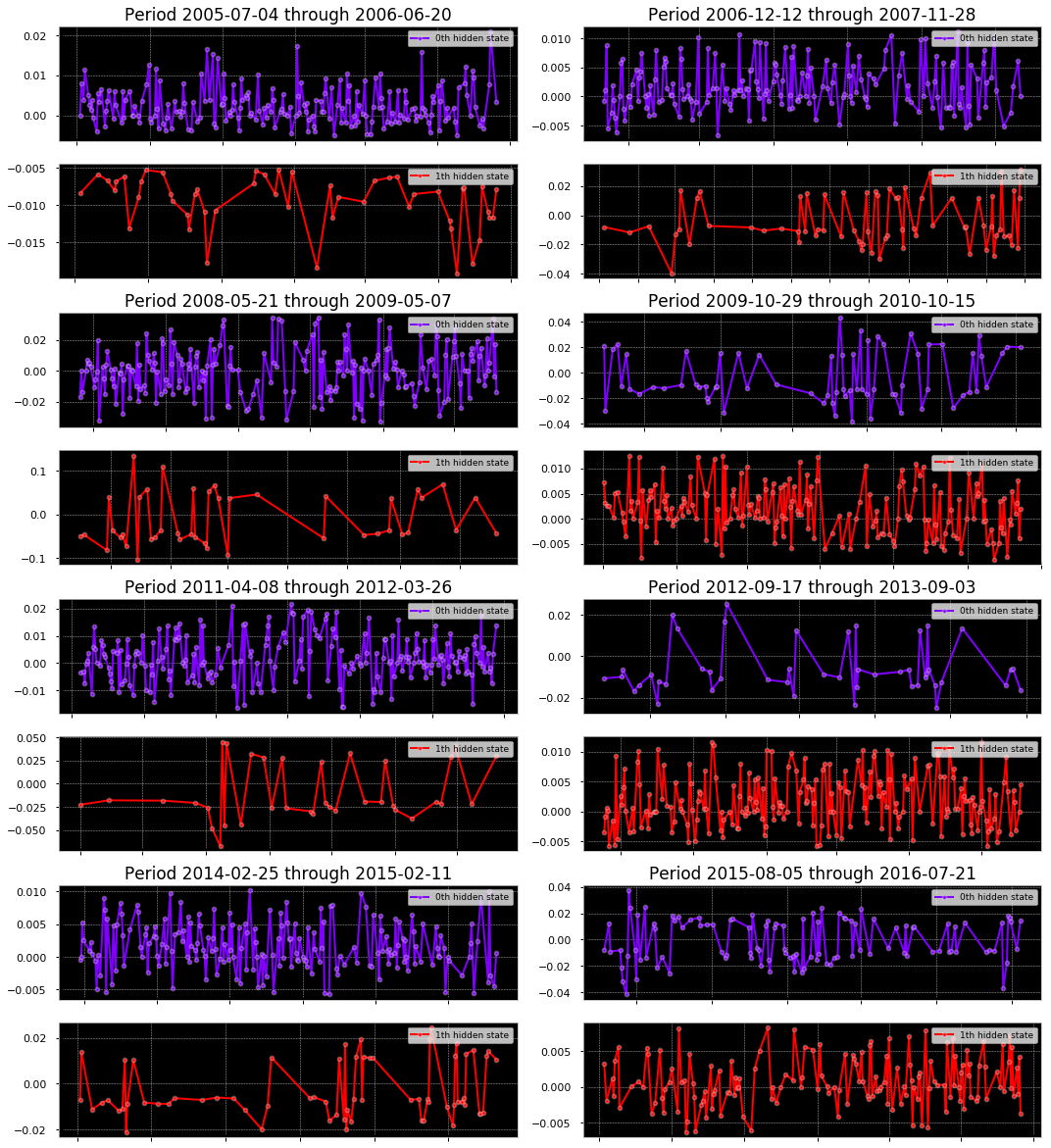We can see how varied the components are across different time periods. Some components are more active while others are rarely active. Additionally notice how some components are clustered over shorter sub periods while others are more evenly distributed.

Next we visualize these relationships further. For each time period, we plot the cumulative returns, color and shape coded to represent the different components. Then we show a boxplot of the aggregate returns over the period. Finally we print out the statistical description of the dataframe using pandas.describe().

In :
def plot_cuml_state(states, state_col=None):
g = (pn.ggplot(states, pn.aes(x='Date',y='mkt_cret', color=f'factor({state_col})'))
+ pn.geom_point(pn.aes(shape=f'factor({state_col})'))
+ pn.geom_hline(yintercept=0., size=1, linetype=':', color='red')
+ pn.scale_y_continuous(breaks=mzb.mpl_breaks(),
labels=mzf.percent_format(),
limits=(states['mkt_cret'].min(), states['mkt_cret'].max()))
+ pn.theme_linedraw()
+ pn.theme(panel_background=pn.element_rect(fill='black'),
axis_text_x=pn.element_text(rotation=50),
text=pn.element_text(size=7))
+ pn.ylab('log returns')
+ pn.ggtitle('Cumulative Log Returns by Hidden State'))
return g

def plot_facet_cuml_states(states, state_col=None):
g = (pn.ggplot(states, pn.aes(x='Date',y='mkt_cret', color=f'factor({state_col})'))
+ pn.geom_point(pn.aes(shape=f'factor({state_col})'))
+ pn.geom_hline(yintercept=0., size=1, linetype=':', color='red')
+ pn.facet_wrap(f'~{state_col}')
+ pn.scale_y_continuous(breaks=mzb.mpl_breaks(),
labels=mzf.percent_format(),
limits=(states['mkt_cret'].min(), states['mkt_cret'].max()))
+ pn.theme_linedraw()
+ pn.theme(panel_background=pn.element_rect(fill='black'),
axis_text_x=pn.element_text(rotation=50),
text=pn.element_text(size=7))
+ pn.ylab('log returns')
+ pn.ggtitle('Cumulative Log Returns by Hidden State'))
return g

def plot_states_boxplot(states, state_col, y_col):
g = (pn.ggplot(states, pn.aes(x=state_col, y=y_col, color=f'factor({state_col})'))
+ pn.geom_boxplot()
+ pn.geom_jitter(alpha=0.5)
+ pn.geom_hline(yintercept=0., size=1, linetype=':', color='red')
+ pn.scale_y_continuous(breaks=mzb.mpl_breaks(),
labels=mzf.percent_format(),
limits=(states[y_col].min(), states[y_col].max()))
+ pn.theme_linedraw()
+ pn.theme(panel_background=pn.element_rect(fill='black'),
axis_text_x=pn.element_text(rotation=30),)
+ pn.ylab('log returns')
+ pn.ggtitle('Log Returns by Hidden State'))
return g


With the plotting functions defined we can loop through the TimeseriesSplit object to generate the plots and descriptive tables for each lookback/training period.

In :
### RUN ###
for i, (train, test) in enumerate(tscv.split(s)):
tmp_train = s.iloc[train].values.reshape(-1,1) # temporary train data
tmp_test = s.iloc[test].values.reshape(-1,1) # temporary test data

gmm = make_gmm(n_components, max_iter)
gmm.fit(tmp_train)

hidden_states_prob = gmm.predict_proba(tmp_train)
hidden_states = gmm.predict(tmp_train)

state_df = (s.iloc[train].to_frame()
.assign(component=pd.Categorical(hidden_states))
.assign(mkt_cret=lambda df: df[sym].cumsum())
.reset_index())

scol = 'component'
g = plot_cuml_state(state_df, state_col=scol)
g1 = plot_facet_cuml_states(state_df, state_col=scol)
g2 = plot_states_boxplot(state_df, scol, sym)
print()
print('*'*77)
print(g)
print(g1)
print(g2)
display(state_df.groupby(scol)[sym].describe().T)

*****************************************************************************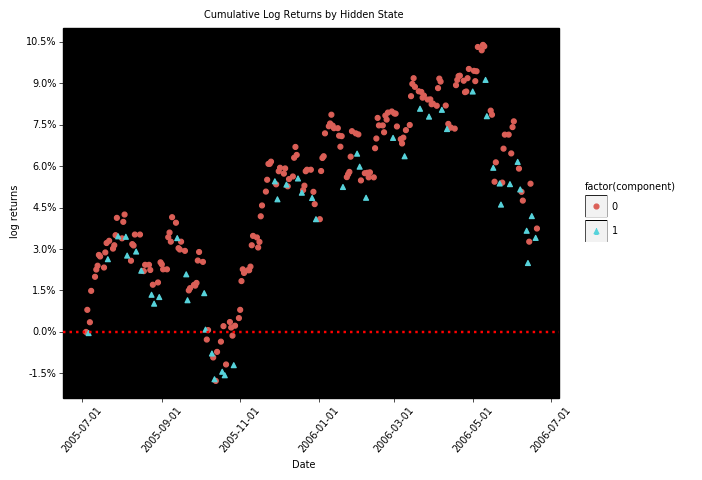<ggplot: (8733357257911)>
`